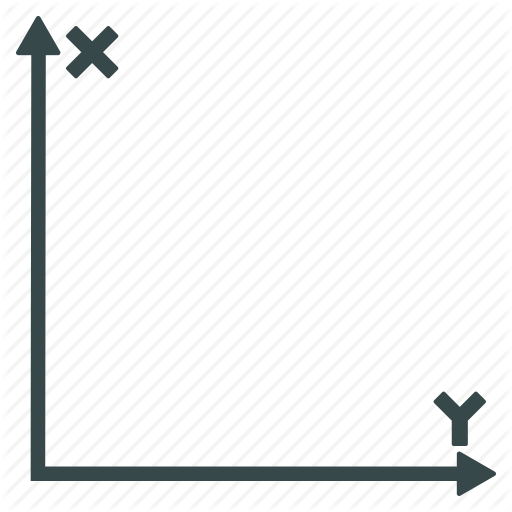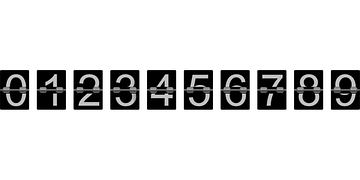Unit Concept Lesson/idea Geometryangles of polygons Lesson where students discover the exterior angles of a polygon using Scratch. Perimeter Lesson where students enter given code to create part of  a shape.  Then figure out what code is missing to complete the shape. tesselations Examples of tessellation projects flip,slide, turn Project incorporating these movements coordinates worksheet and plan for teaching coordinates in Scratch measurement Lesson in which students use a Beebot to predict then measure the distance across their desk.  Lesson explanation directions Lesson in which students use a Beebot to give precise directions to their treasure. Blog post Numberprobability Scratch programs to teach probability Equivalent Fractions Scratch program to help students visualize fractionsNote: A web based version Eqv. Fractions. by GLUA.fractions worksheetvideo of my class exploring this concept prime factor challenge Project Page on Scratch Teaching Fractions through Music Blog post on a lesson which has students write code to create their own music and teaches students concepts of fractions and decimals Student reflection sheet Counting and cardinality Scratch Jr project makes a game for guessing how many cats are on the screen. The player will know whether he or she has tapped on the correct answer by reading the message that appears.  printable project page counting Count forward and backward by any number using Beebots Lesson explanation      video Addition Addition and coding game with Beebots:  video of lesson explanation Problem SolvingBinary Search-Using Logic and Math The best guess is always the midpoint. Simple calculation. Calculates the cost of a trip using a simple program.Cost of trip.